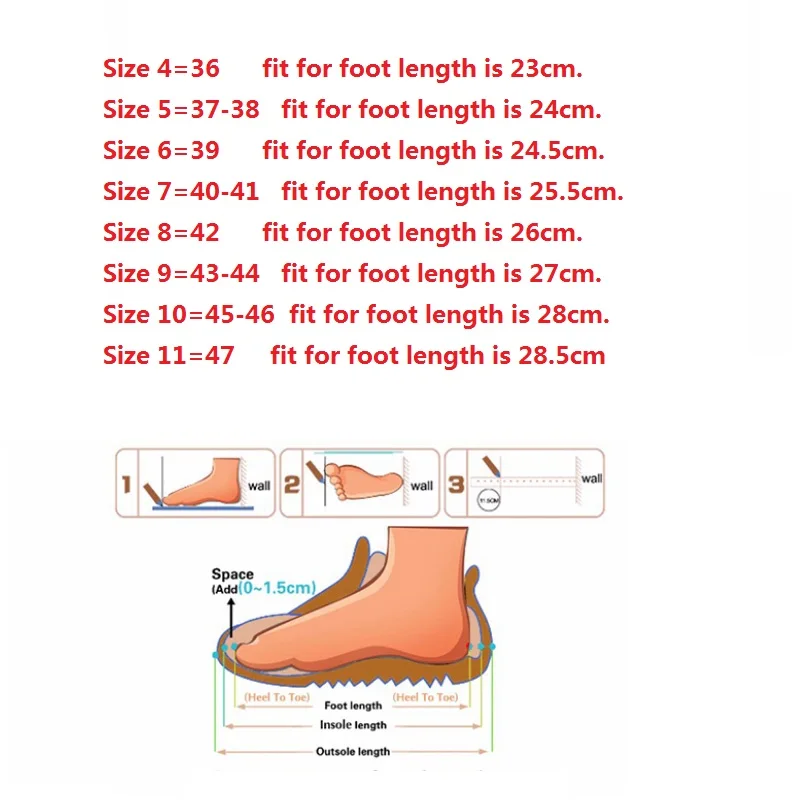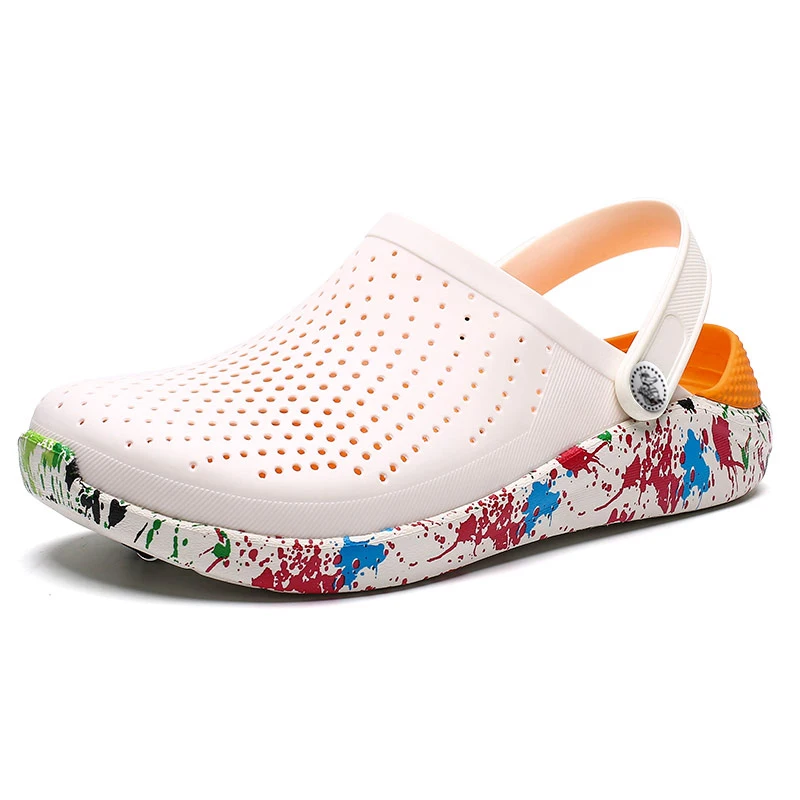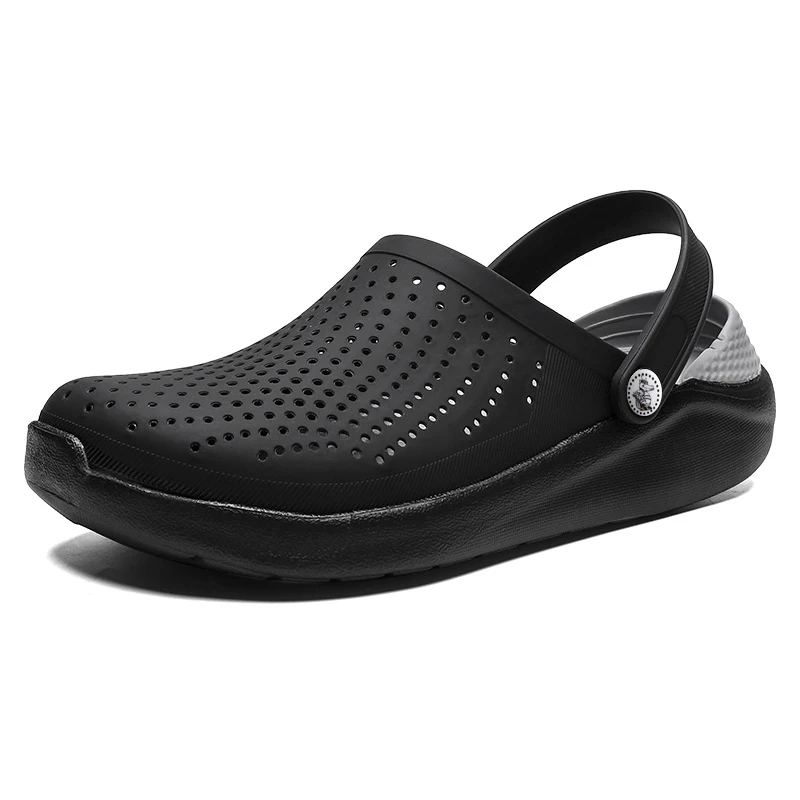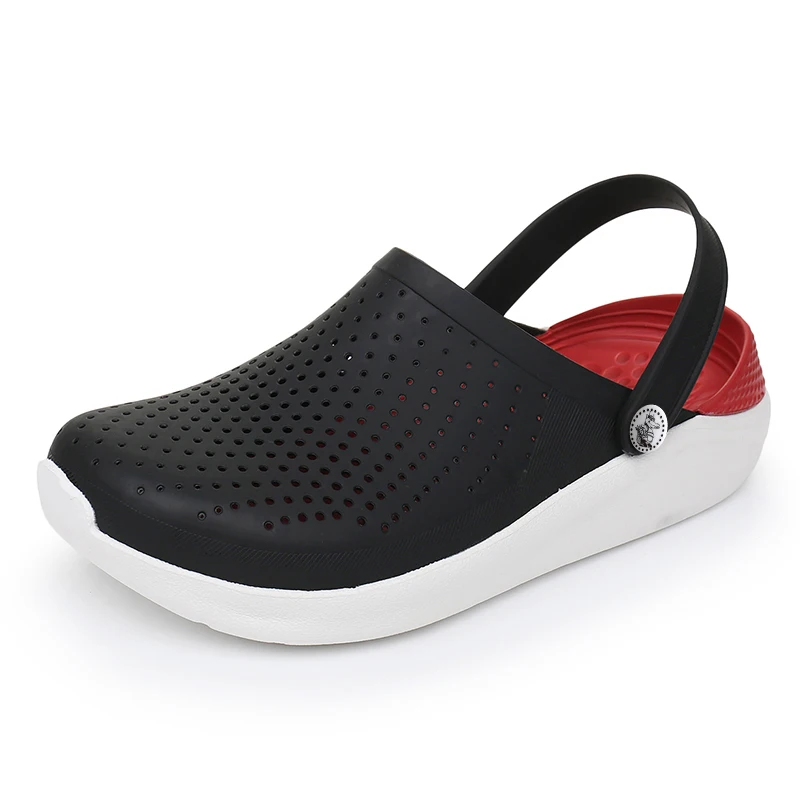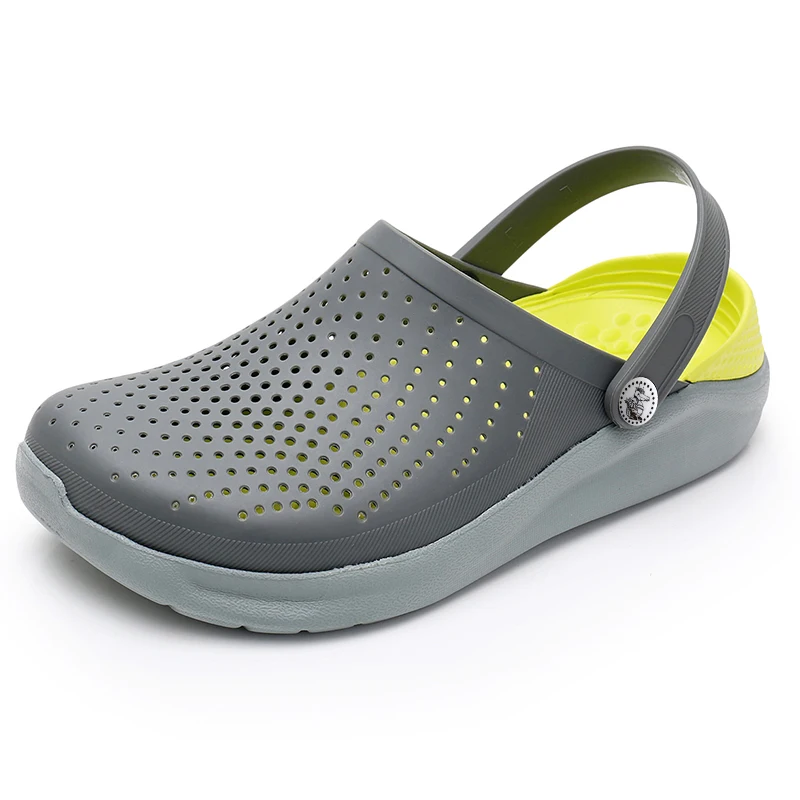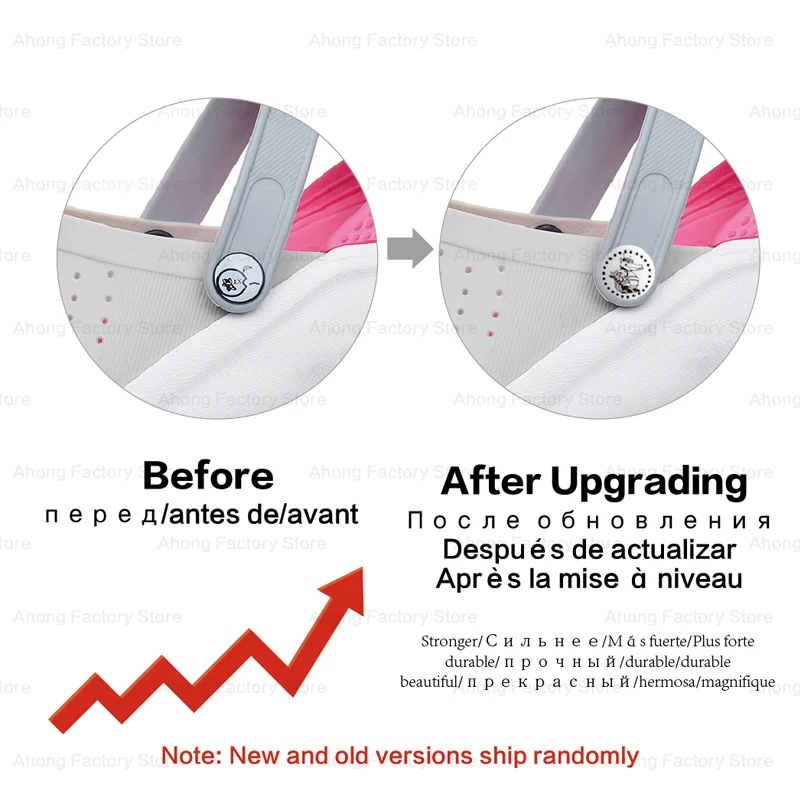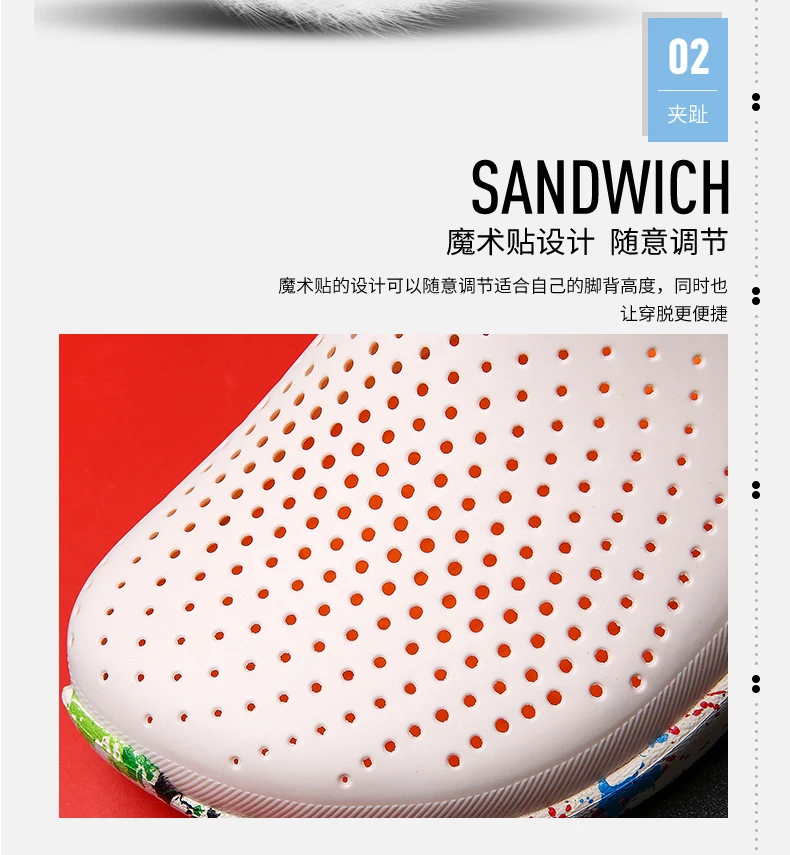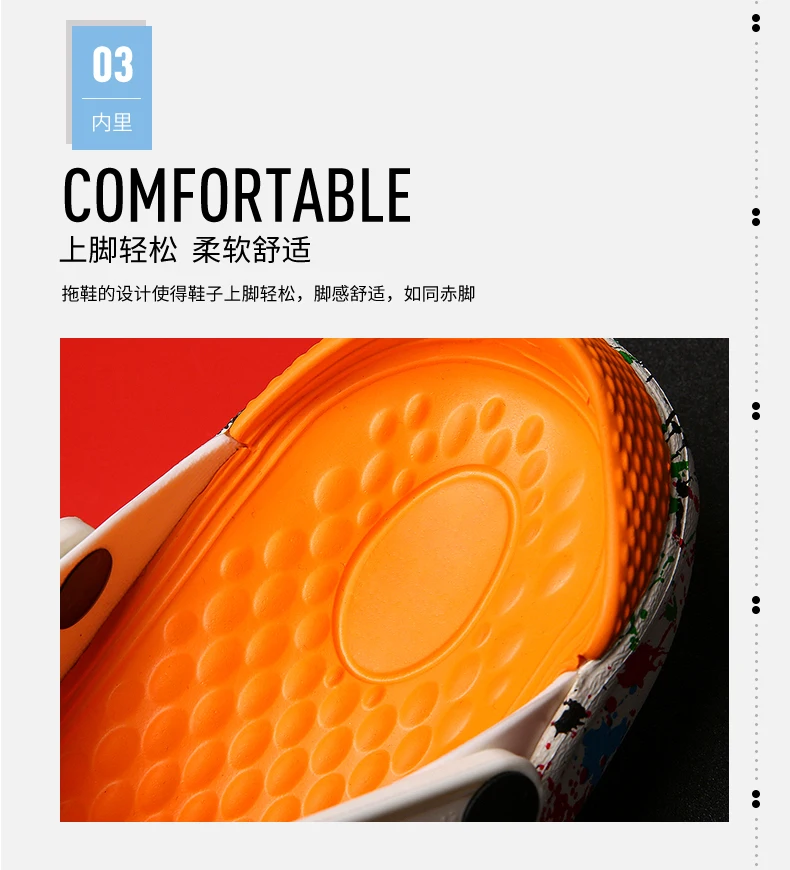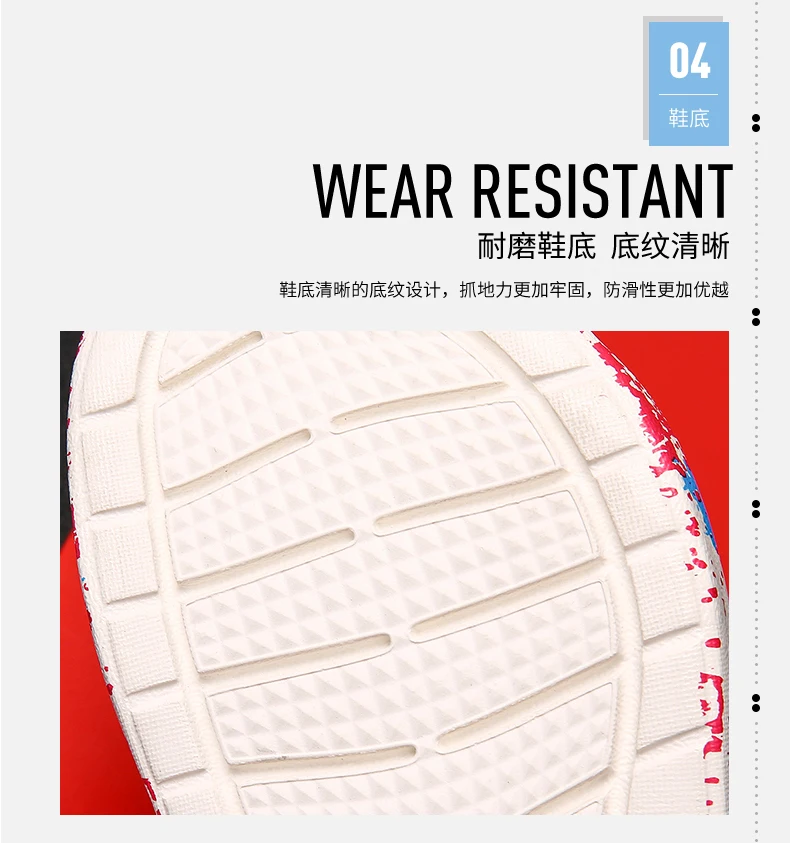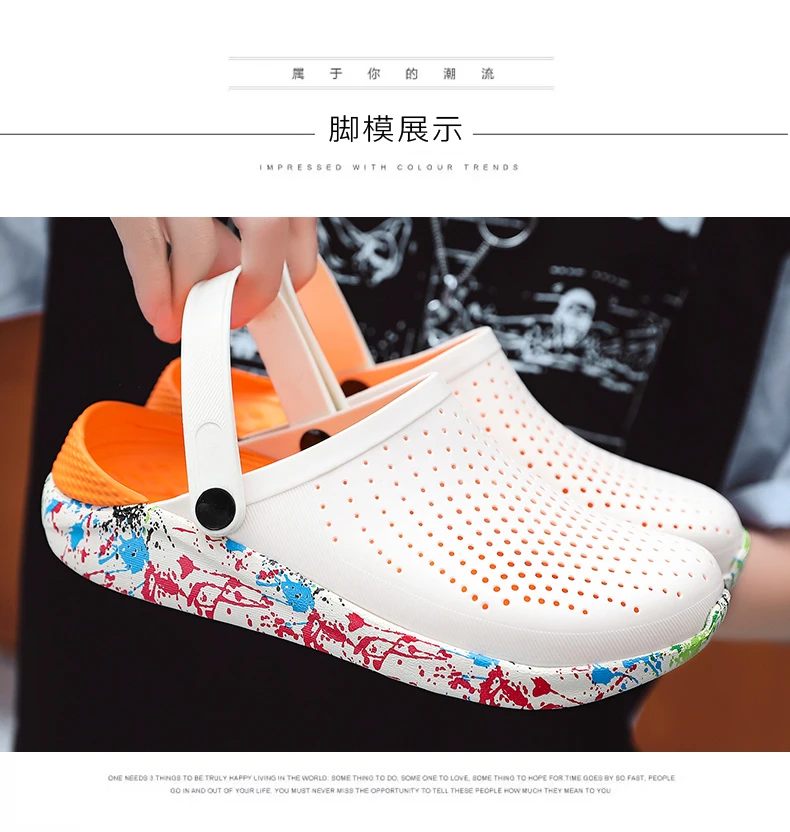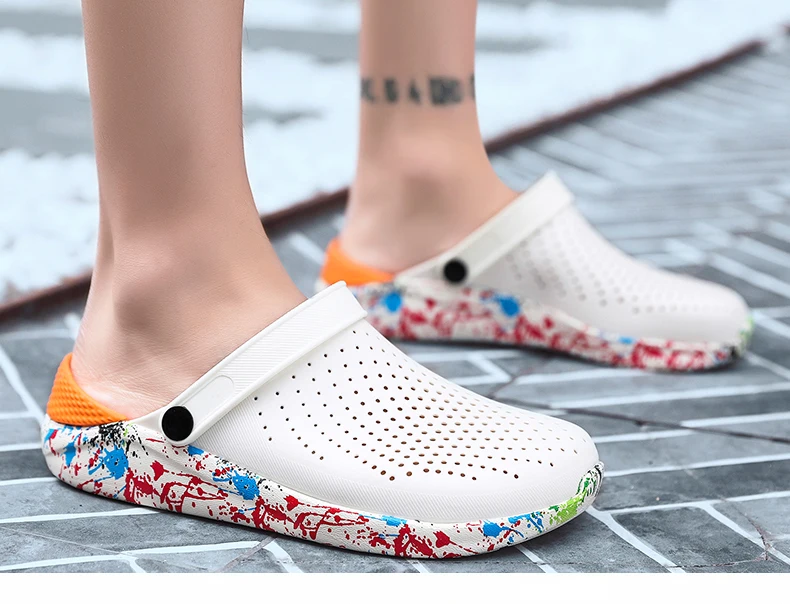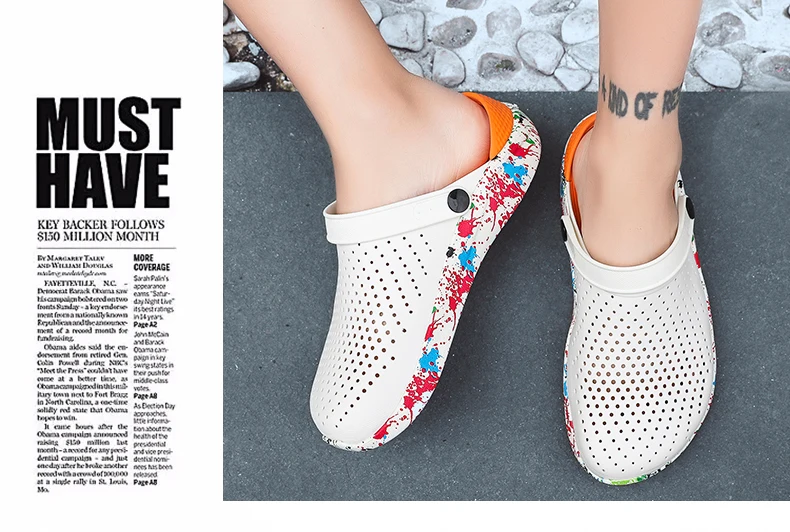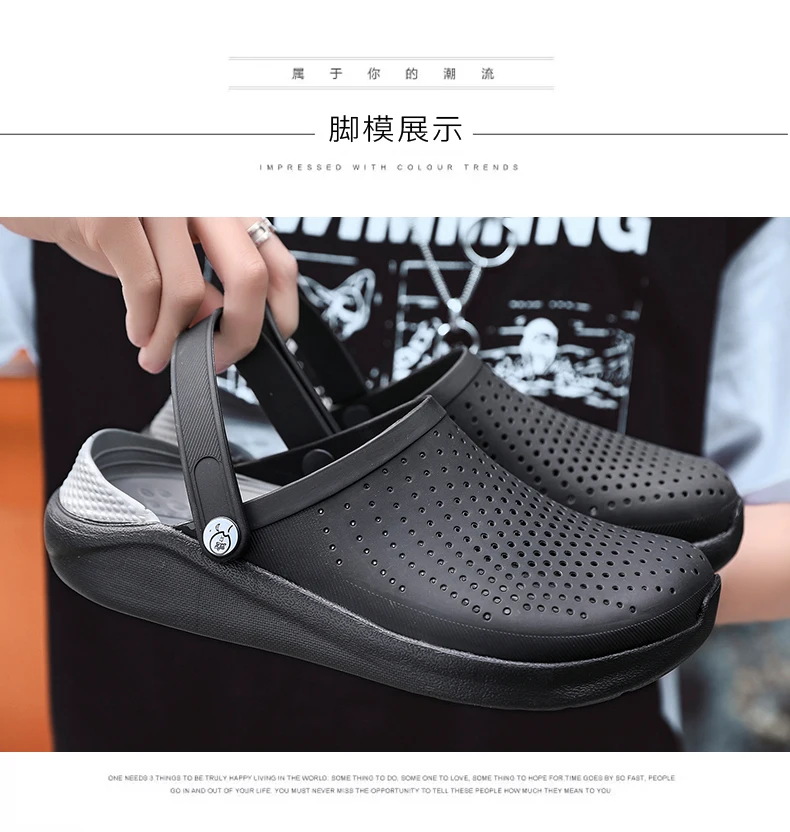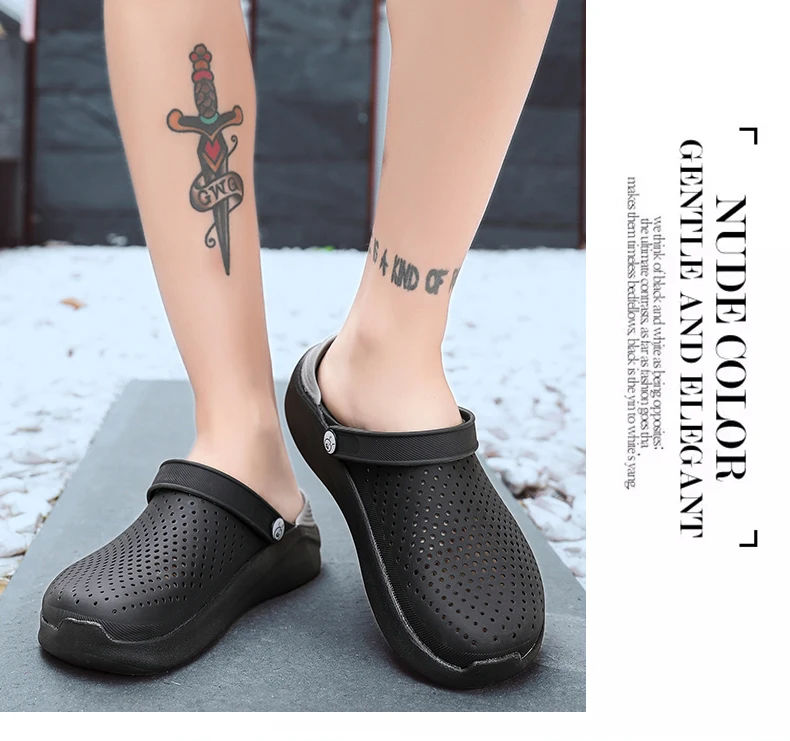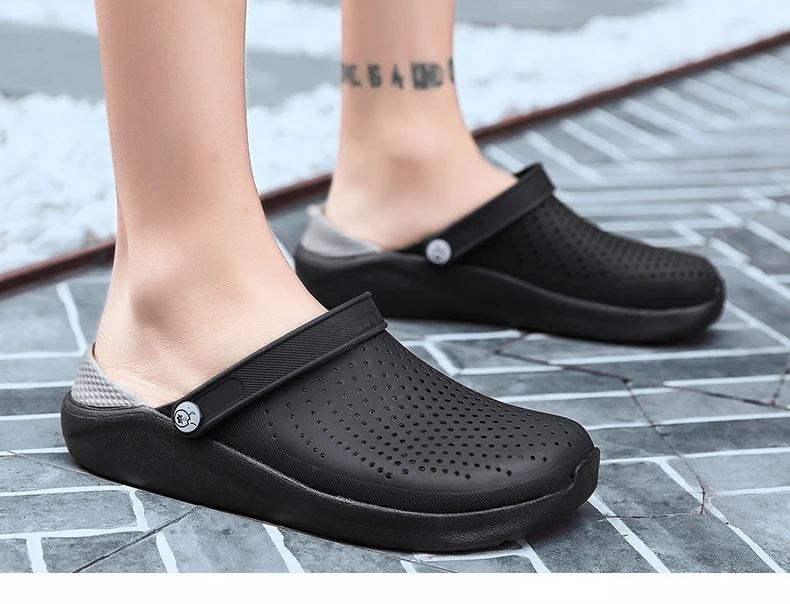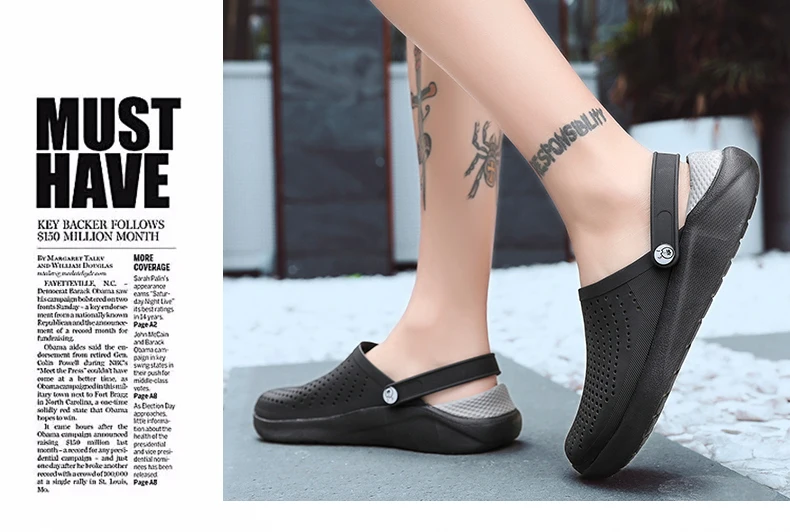# 2020 men's summer ripped sandals for men

Regular price \$19.00Size 4=36 fit for foot length is 23cm.
Size 5=37-38 fit for foot length is 24cm.
Size 6=39 fit for foot length is 24.5cm.
Size 7=40-41 fit for foot length is 25.5cm.
Size 8=42 fit for foot length is 26cm.
Size 9=43-44 fit for foot length is 27cm.
Size 10=45-46 fit for foot length is 28cm.
Size 11=47 fit for foot length is 28.5cm